• mopso多目标粒子群算法 python源码 粒子群速度位置更新 pareto最优解集 外部存档 拥挤度计算
• 粒子群算法Python实现
根据适应度值来计算局部最优和全局最优。前者表示迭代以来，该粒子的最优解；后者是局部最优的最优。
局部最优对应了局部学习率，全局最优对应了全局学习率，用来计算更新速度。更新速度负责对粒子位置进行更新。
粒子的速度有个范围，例子的位置也有个范围。
寻找最优解的函数为f(x)=sin⁡x2+y2x2+y2+ecos⁡2πx+cos2πy2−2.71289
f(x) = \frac{\sin\sqrt{x^2+y^2}}{\sqrt{x^2+y^2}} +e^{\frac{\cos2\pi x +cos2\pi y}{2}}-2.71289

其函数图像如下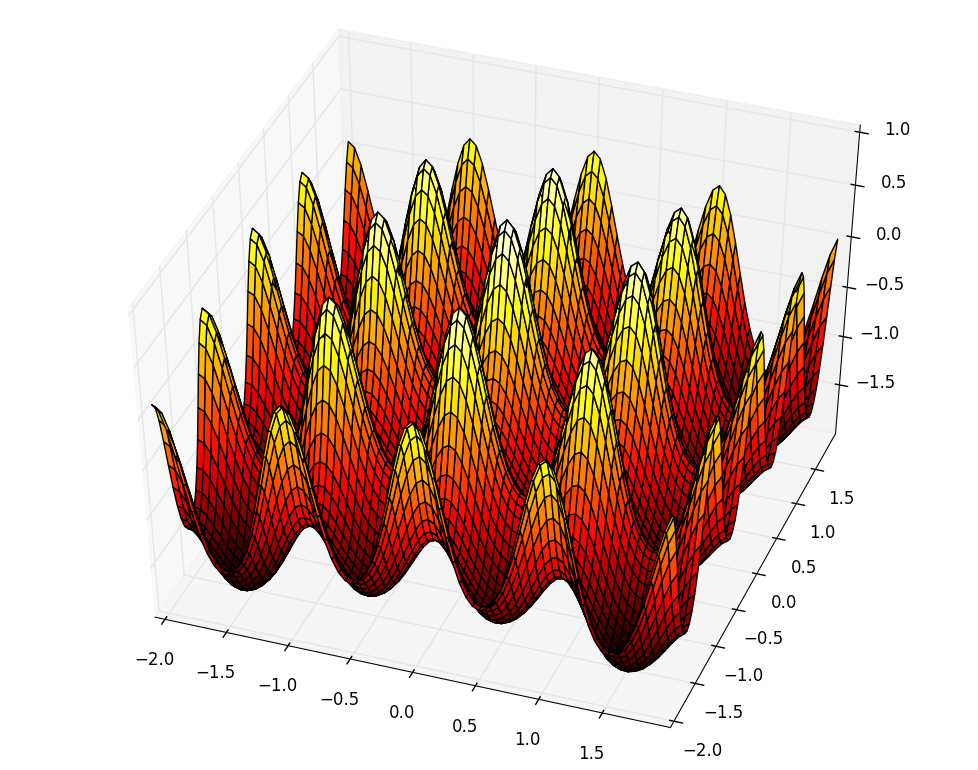该三维图像代码如下：
from mpl_toolkits.mplot3d import Axes3D

fig = figure()
ax = Axes3D(fig)
X = np.arange(-2, 2, 0.05)
Y = np.arange(-2, 2, 0.05)
X, Y = np.meshgrid(X, Y)
Z = np.sin(np.sqrt(X**2+Y**2))/np.sqrt(X**2+Y**2)+np.exp((np.cos(2*np.pi*X)+np.cos(2*np.pi*Y))/2)-2.71289
ax.plot_surface(X, Y, Z, rstride=1, cstride=1, cmap='hot')

粒子群算法Python实现源码：
import numpy as np

def getweight():
# 惯性权重
weight = 1
return weight

def getlearningrate():
# 分别是粒子的个体和社会的学习因子，也称为加速常数
lr = (0.49445,1.49445)
return lr

def getmaxgen():
# 最大迭代次数
maxgen = 300
return maxgen

def getsizepop():
# 种群规模
sizepop = 20
return sizepop

def getrangepop():
# 粒子的位置的范围限制,x、y方向的限制相同
rangepop = (-2,2)
return rangepop

def getrangespeed():
# 粒子的速度范围限制
rangespeed = (-0.5,0.5)
return rangespeed

def func(x):
# x输入粒子位置
# y 粒子适应度值
if (x==0)&(x==0):
y = np.exp((np.cos(2*np.pi*x)+np.cos(2*np.pi*x))/2)-2.71289
else:
y = np.sin(np.sqrt(x**2+x**2))/np.sqrt(x**2+x**2)+np.exp((np.cos(2*np.pi*x)+np.cos(2*np.pi*x))/2)-2.71289
return y

def initpopvfit(sizepop):
pop = np.zeros((sizepop,2))
v = np.zeros((sizepop,2))
fitness = np.zeros(sizepop)

for i in range(sizepop):
pop[i] = [(np.random.rand()-0.5)*rangepop*2,(np.random.rand()-0.5)*rangepop*2]
v[i] = [(np.random.rand()-0.5)*rangepop*2,(np.random.rand()-0.5)*rangepop*2]
fitness[i] = func(pop[i])
return pop,v,fitness

def getinitbest(fitness,pop):
# 群体最优的粒子位置及其适应度值
gbestpop,gbestfitness = pop[fitness.argmax()].copy(),fitness.max()
#个体最优的粒子位置及其适应度值,使用copy()使得对pop的改变不影响pbestpop，pbestfitness类似
pbestpop,pbestfitness = pop.copy(),fitness.copy()

return gbestpop,gbestfitness,pbestpop,pbestfitness

w = getweight()
lr = getlearningrate()
maxgen = getmaxgen()
sizepop = getsizepop()
rangepop = getrangepop()
rangespeed = getrangespeed()

pop,v,fitness = initpopvfit(sizepop)
gbestpop,gbestfitness,pbestpop,pbestfitness = getinitbest(fitness,pop)

result = np.zeros(maxgen)
for i in range(maxgen):

#速度更新
for j in range(sizepop):
v[j] += lr*np.random.rand()*(pbestpop[j]-pop[j])+lr*np.random.rand()*(gbestpop-pop[j])
v[v<rangespeed] = rangespeed
v[v>rangespeed] = rangespeed

#粒子位置更新
for j in range(sizepop):
pop[j] += 0.5*v[j]
pop[pop<rangepop] = rangepop
pop[pop>rangepop] = rangepop

#适应度更新
for j in range(sizepop):
fitness[j] = func(pop[j])

for j in range(sizepop):
if fitness[j] > pbestfitness[j]:
pbestfitness[j] = fitness[j]
pbestpop[j] = pop[j].copy()

if pbestfitness.max() > gbestfitness :
gbestfitness = pbestfitness.max()
gbestpop = pop[pbestfitness.argmax()].copy()

result[i] = gbestfitness

plot(result)

全局最优解随迭代次数增加的变化情况如下图所示：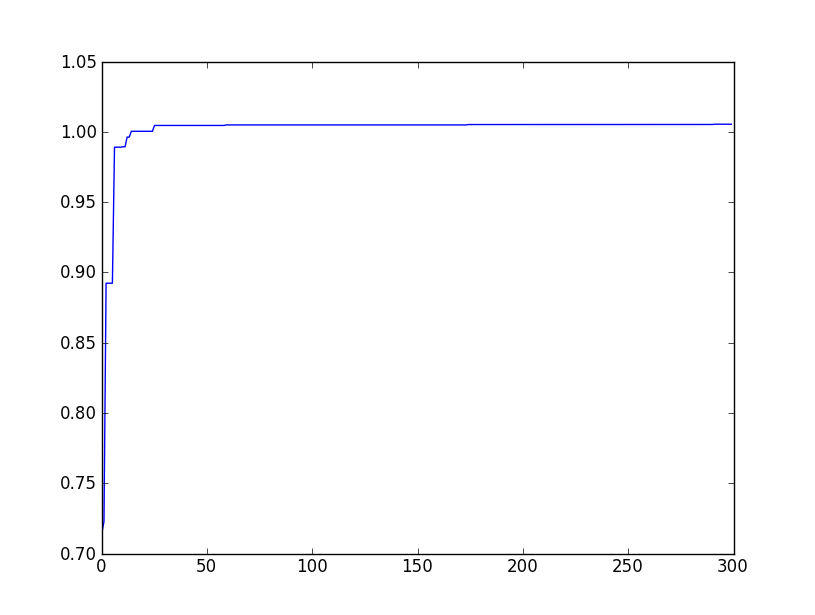展开全文• 粒子群算法Python实现。除此之外，还有这些算法的集合：差分进化算法、遗传算法、粒子群算法、模拟退火算法、蚁群算法、免疫优化算法、鱼群算法
• 粒子群算法，也称粒子群优化算法或鸟群觅食算法（Particle Swarm Optimization），缩写为 PSO， 是近年来由J. Kennedy和R. C. Eberhart等  开发的一种新的进化算法(Evolutionary Algorithm - EA)。PSO 算法属于...
• 粒子群算法 模拟退火算法 蚁群算法 免疫优化算法 人工鱼群算法 项目链接： https://github.com/zhangxiao123qqq/scikit-opt 原作者链接： https://github.com/guofei9987/scikit-opt 黑盒实现，即拿即用！不要太爽...
工具包
在GitHub上找到一个开源的项目，并对其进行了更新，提交了一些小bug，它实现了数学建模中常用的算法包括：

差分进化算法
遗传算法
粒子群算法
模拟退火算法
蚁群算法
免疫优化算法
人工鱼群算法

项目链接：
https://github.com/zhangxiao123qqq/scikit-opt
原作者链接：
https://github.com/guofei9987/scikit-opt
黑盒实现，即拿即用！不要太爽！！
提示：项目中使用等help()命令可直接获得参数说明。
#遗传算法帮助
help(GA)
#模拟退火算法帮助
help(SA)

算法使用方式见：
中文使用文档
Demo演示:
demo_ga
import numpy as np

def schaffer(p):
'''
This function has plenty of local minimum, with strong shocks
global minimum at (0,0) with value 0
'''
x1, x2 = p
x = np.square(x1) + np.square(x2)
return 0.5 + (np.square(np.sin(x)) - 0.5) / np.square(1 + 0.001 * x)

# %%
from sko.GA import GA

ga = GA(func=schaffer, n_dim=2, size_pop=50, max_iter=800, lb=[-1, -1], ub=[1, 1], precision=1e-7)
best_x, best_y = ga.run()
print('best_x:', best_x, '\n', 'best_y:', best_y)

# %% Plot the result
import pandas as pd
import matplotlib.pyplot as plt

Y_history = pd.DataFrame(ga.all_history_Y)
fig, ax = plt.subplots(2, 1)
ax.plot(Y_history.index, Y_history.values, '.', color='red')
Y_history.min(axis=1).cummin().plot(kind='line')
plt.show()



展开全文数学建模
• 基于Python实现的粒子群算法，上传上来方便大家交流学习，算法实现了最基础的粒子群算法，并附带简单的注释，大家可以根据自己的需要进行修改。粒子群算法是群智能一种，是基于对鸟群觅食行为的研究和模拟而来的。...
• 粒子群算法python实现 这里的目标函数为rastrigin函数，图像如下所示： 本算法主要分为三个部分： 1 .将粒子群算法的参数初始化 2 .初始化种群，并初始化个体最优pbest与全局最优gbset 3 .进行循环更新个体 #...
粒子群算法的python实现
这里的目标函数为rastrigin函数，图像如下所示：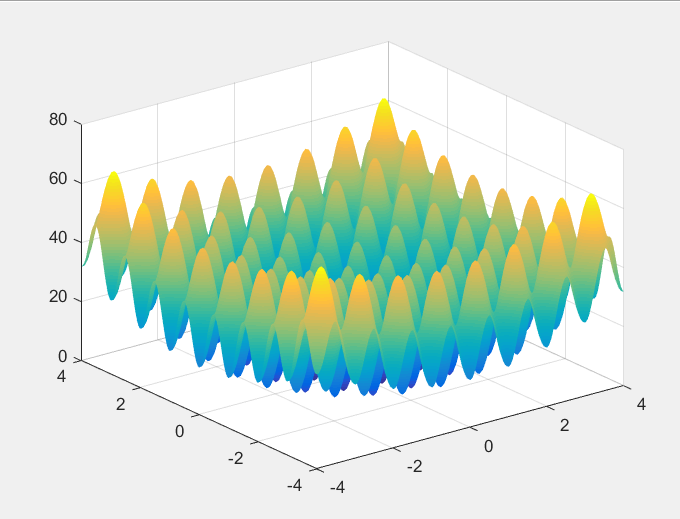本算法主要分为三个部分：

1 .将粒子群算法的参数初始化
2 .初始化种群，并初始化个体最优pbest与全局最优gbset
3 .进行循环更新个体

#python编写pso算法
import numpy as np
import matplotlib.pyplot as plt
import random

class PSO_model:
def __init__(self,w,c1,c2,r1,r2,N,D,M):
"""
w：惯性权值
c1:学习因子1
c2:学习因子2
r1:学习率1
r2:学习率2
N:初始化种群数量个数
D：搜索空间维度
M：迭代的最大次数

"""
self.w = w
self.c1=c1
self.c2=c2
self.r1=r1
self.r2=r2
self.N=N
self.D=D
self.M=M
self.x=np.zeros((self.N,self.D))#粒子的初始位置
self.v=np.zeros((self.N,self.D))#粒子的初始速度
self.pbest=np.zeros((self.N,self.D))#个体最优值初始化
self.gbest=np.zeros((1,self.D))#种群最优值
self.p_fit=np.zeros(self.N)
self.fit=1e8#初始化全局最优适应度10^8

#目标函数，也是适应度函数（求最小化问题）
def function(self,x):
A = 10
x1=x
x2=x
Z = 2 * A + x1 ** 2 - A * np.cos(2 * np.pi * x1) + x2 ** 2 - A * np.cos(2 * np.pi * x2)
#print("z",Z)
return Z

#初始化种群（初始化了个体最优值和种群最优值）
def init_pop(self):
for i in range(self.N):
for j in range(self.D):
self.x[i][j] = random.random()
self.v[i][j] = random.random()
self.pbest[i] = self.x[i]#初始化个体的最优值
aim=self.function(self.x[i])#计算个体的适应度值
print("aim",aim)
self.p_fit[i]=aim#初始化个体的最优位置
if aim < self.fit:  # 对个体适应度进行比较，计算出最优的种群适应度
self.fit = aim
self.gbest = self.x[i]#谁小谁最优

#更新粒子的位置与速度
def update(self):
fitness=[]
for t in range(self.M):#在迭代次数M内进行循环
for i in range(self.N):#对所有种群进行一次循环
aim=self.function(self.x[i])#计算一次目标函数的适应度
if aim<self.p_fit[i]:#比较适应度大小，将小的负值给个体最优
self.p_fit[i]=aim
self.pbest[i]=self.x[i]
if self.p_fit[i]<self.fit:#如果是个体最优再将和全体最优进行对比
self.gbest=self.x[i]
self.fit = self.p_fit[i]
for i in range(self.N):#更新粒子的速度和位置
self.v[i]=self.w*self.v[i]+self.c1*self.r1*(self.pbest[i]-self.x[i])+ self.c2*self.r2*(self.gbest-self.x[i])
self.x[i]=self.x[i]+self.v[i]
fitness.append(self.fit)#将每一次的结果保留在数组中
print(self.x,end='')
print(self.fit)  # 输出最优值
return fitness

if __name__ == '__main__':
# w,c1,c2,r1,r2,N,D,M参数初始化
w=random.random()
c1=c2=2#一般设置为2
r1=0.7
r2=0.5
N=20
D=2
M=100
pso_object=PSO_model(w,c1,c2,r1,r2,N,D,M)#设置初始权值
pso_object.init_pop()
fitness=pso_object.update()
#画图
plt.figure()
plt.plot(fitness)
plt.show()


结果如下：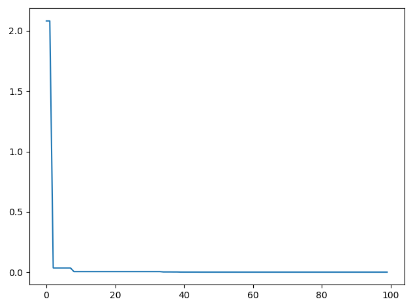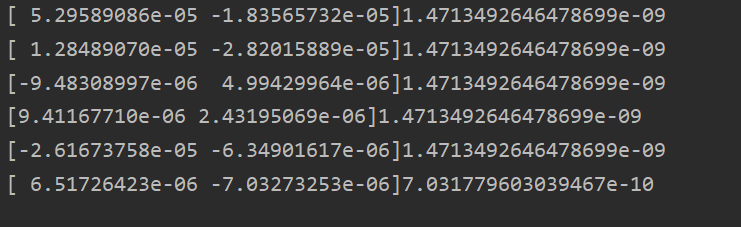参考：
https://blog.csdn.net/winycg/article/details/79120154


展开全文• 1.粒子群算法简介 粒子群算法的思想源于对鸟类捕食行为的研究，模拟鸟集群飞行觅食的行为，鸟之间通过集体的协作使群体达到最优目的。 设想这样的一个场景，一群鸟在随机的搜索食物，在某块区域里有一块食物， 2....
目录1.算法简介2.算法流程3.算法示例4.算法实现5.算法应用
1.算法简介
粒子群算法的思想源于对鸟类捕食行为的研究，模拟鸟集群飞行觅食的行为，鸟之间通过集体的协作使群体达到最优目的。
设想这样的一个场景，一群鸟在随机的搜索食物，在某块区域里有一块食物，所有的鸟都不知道食物在哪里，但是他们可以感受到当前的位置离食物还有多远，此时找到食物的最优策略是什么？答案是：搜寻目前离食物最近的鸟的周围区域，根据自己的飞行经验判断食物的所在。
PSO正是从这种模型中得到了启发：
1.每个寻优问题的解都被想象成一只鸟，称为‘粒子’，所有的粒子都在一个D维空间进行搜索。
2.所有的粒子都由一个fitness function确定适应值以判断目前位置的好坏。
3.每一个粒子都被赋予记忆功能，能记住所搜寻到的最佳位置
4.每一个粒子还有一个速度以决定飞行的距离和方向。这个速度根据本身的飞行经验及同伴的飞行经验进行动态调整。
5.可以看出粒子群算法的基础在于信息的社会共享
D维空间中，有N个粒子；
粒子i位置：xi=(xi1,xi2,…xiD)，将xi代入适应函数f(xi)求适应值；
粒子i速度：vi=(vi1,vi2,…viD)
粒子i个体经历过的最好位置：pbesti=(pi1,pi2,…piD)
种群所经历过的最好位置：gbest=(g1,g2,…gD)
通常，在第d（1≤d≤D）维的位置变化范围限定在($X_{min,d},X_{max,d}$)内，
速度变化范围限定在($-V_{max,d},V_{max,d}$) 内（即在迭代中若
超出了边界值，则该维的速度或位置被限制为该维最大速度或边界
位置）
速度及位置的更新公式
有了上面的了解后，一个很关键的问题就是粒子的速度以及位置如何更新？
粒子i的第d维速度更新公式：粒子i的第d维位置更新公式$v_{id}^k$：第k次迭代粒子i的飞行速度矢量的第d维分量
$x_{id}^k$：第k次迭代粒子i位置矢量的第d维分量
c1,c2：加速度常数，调节学习最大步长（超参数）
r1,r2：两个随机函数，取值范围[0,1],以增加随机性
w：惯性权重，非负数，调节对解空间的搜索范围。（超参数）
可以看出pso算法一共含有3个超参数。
对于粒子速度更新公式这里多介绍一些：其速度更新公式包含三个部分：
第一部分为粒子先前的速度
第二部分为‘认知’部分，表示粒子本身的思考，可理解为粒子i当前位置与自己最好位置之间的距离
第三部分为‘社会部分’，表示粒子间的信息共享和合作，可理解为粒子i当前位置与群体最好位置之间的距离。
如下图所示，粒子其运动的过程受以上三个方面的影响：2.算法流程

Initial：
初始化粒子群体（群体规模为n），包括随机位置和速度。
Evaluation：
根据fitness function ，评价每个粒子的适应度。
Find the Pbest：
对每个粒子，将其当前适应值与其个体历史最佳位置（pbest）对应的适应值做比较，如果当前的适应值更高，则将用当前位置更新历史最佳位置pbest。
Find the Gbest：
对每个粒子，将其当前适应值与全局最佳位置（gbest）对应的适应值做比较，如果当前的适应值更高，则将用当前粒子的位置更新全局最佳位置gbest。
Update the Velocity：
根据公式更新每个粒子的速度与位置。
如未满足结束条件，则返回步骤2
通常算法达到最大迭代次数或者最佳适应度值的增量小于某个给定的阈值时算法停止。

该算法的流程图如下：3.算法示例4.算法实现
以上面的例子为例，该算法的实现如下，如果需要优化其他问题，只需要调整下fitness function即可。
import numpy as np
import matplotlib.pyplot as plt
from mpl_toolkits.mplot3d import Axes3D

def fit_fun(x):  # 适应函数
return sum(100.0 * (x[1:] - x[:-1] ** 2.0) ** 2.0 + (1 - x[:-1]) ** 2.0)

class Particle:
# 初始化
def __init__(self, x_max, max_vel, dim):
self.__pos = np.random.uniform(-x_max, x_max, (1, dim))  # 粒子的位置
self.__vel = np.random.uniform(-max_vel, max_vel, (1, dim))  # 粒子的速度
self.__bestPos = np.zeros((1, dim))  # 粒子最好的位置
self.__fitnessValue = fit_fun(self.__pos)  # 适应度函数值

def set_pos(self, value):
self.__pos = value

def get_pos(self):
return self.__pos

def set_best_pos(self, value):
self.__bestPos = value

def get_best_pos(self):
return self.__bestPos

def set_vel(self, value):
self.__vel = value

def get_vel(self):
return self.__vel

def set_fitness_value(self, value):
self.__fitnessValue = value

def get_fitness_value(self):
return self.__fitnessValue

class PSO:
def __init__(self, dim, size, iter_num, x_max, max_vel, tol, best_fitness_value=float('Inf'), C1=2, C2=2, W=1):
self.C1 = C1
self.C2 = C2
self.W = W
self.dim = dim  # 粒子的维度
self.size = size  # 粒子个数
self.iter_num = iter_num  # 迭代次数
self.x_max = x_max
self.max_vel = max_vel  # 粒子最大速度
self.tol = tol  # 截至条件
self.best_fitness_value = best_fitness_value
self.best_position = np.zeros((1, dim))  # 种群最优位置
self.fitness_val_list = []  # 每次迭代最优适应值

# 对种群进行初始化
self.Particle_list = [Particle(self.x_max, self.max_vel, self.dim) for i in range(self.size)]

def set_bestFitnessValue(self, value):
self.best_fitness_value = value

def get_bestFitnessValue(self):
return self.best_fitness_value

def set_bestPosition(self, value):
self.best_position = value

def get_bestPosition(self):
return self.best_position

# 更新速度
def update_vel(self, part):
vel_value = self.W * part.get_vel() + self.C1 * np.random.rand() * (part.get_best_pos() - part.get_pos()) \
+ self.C2 * np.random.rand() * (self.get_bestPosition() - part.get_pos())
vel_value[vel_value > self.max_vel] = self.max_vel
vel_value[vel_value < -self.max_vel] = -self.max_vel
part.set_vel(vel_value)

# 更新位置
def update_pos(self, part):
pos_value = part.get_pos() + part.get_vel()
part.set_pos(pos_value)
value = fit_fun(part.get_pos())
if value < part.get_fitness_value():
part.set_fitness_value(value)
part.set_best_pos(pos_value)
if value < self.get_bestFitnessValue():
self.set_bestFitnessValue(value)
self.set_bestPosition(pos_value)

def update_ndim(self):

for i in range(self.iter_num):
for part in self.Particle_list:
self.update_vel(part)  # 更新速度
self.update_pos(part)  # 更新位置
self.fitness_val_list.append(self.get_bestFitnessValue())  # 每次迭代完把当前的最优适应度存到列表
print('第{}次最佳适应值为{}'.format(i, self.get_bestFitnessValue()))
if self.get_bestFitnessValue() < self.tol:
break

return self.fitness_val_list, self.get_bestPosition()

if __name__ == '__main__':
# test 香蕉函数
pso = PSO(4, 5, 10000, 30, 60, 1e-4, C1=2, C2=2, W=1)
fit_var_list, best_pos = pso.update_ndim()
print("最优位置:" + str(best_pos))
print("最优解:" + str(fit_var_list[-1]))
plt.plot(range(len(fit_var_list)), fit_var_list, alpha=0.5)


5.算法应用
注意该算法在解决具体问题时需要注意以下几点：
1.种群大小m
m很小很容易陷入局部最优，m很大，pso的优化能力很好，当种群数目增长至一定水平时，再增长将不再有显著的作用。
2.权重因子
对于粒子的速度更新的三部分：
a. 惯性因子w=1表示基本的粒子群算法，w=0表示失去对粒子本身的速度记忆。
b. 自我认知部分的学习因子c1=0表示无私型的粒子群算法，只有社会，没有自我，这样会使群体丧失多样性，从而容易导致陷入局部最优而无法跳出。
c. 社会经验部分的学习因子c2=0表示自我型的粒子群算法，只有自我没有社会，这样导致没有信息的社会共享，算法收敛速度缓慢。
这三个参数的选择非常重要，如何调整这三个参数使算法避免早熟又可以比较快的收敛，对于解决实际问题意义较大。
3.最大速度
速度限制的作用为：维护算法的探索能力与开发能力的平衡。
$V_m$较大时，探索能力强，但是粒子容易飞过最优解
$V_m$较小时，开发能力强，但是容易陷入局部最优解
$V_m$一般设定为每维变量变化范围的10%~20%
4.停止准则
a.最大迭代次数
b.可以接受的满意解（通过fitness function判断是否满意）
5.粒子空间的初始化
较好地选择粒子的初始化空间，将大大缩短收敛时间．初始化空间根据具体问题的不同而不同，根据具体问题进行设定．   该算法为数不多的关键参数的设置却对算法的精度和效率有
着显著影响．
6.线性递减权值（未测）$w_{max}$最大惯性权重，$w_{min}$最小惯性权重，run当前迭代次数，$run_{max}$为算法迭代总次数
较大的w有较好的全局收敛能力，较小的w则有较强的局部收敛能力。因此，随着迭代次数的增加，惯性权重w应不断减少，从而使得粒子群算法在初期具有较强的全局收敛能力，而晚期具有较强的局部收敛能力。
7.收缩因子法（未测）1999年，Clerc引入收缩因子以保证算法的收敛性，即速度更新公式改为上式，其中，收缩因子K为受φ1 φ2 限制的w。φ1 φ2是需要预先设定的模型参数。收缩因子法控制系统行为最终收敛，且可以有效搜索不同区域，该法能得到较高质量的解。
8.算法的邻域拓扑结构（未测）
粒子群算法的邻域拓扑结构包括两种，一种是将群体内所有个体都作为粒子的邻域，另一种是只将群体中的部分个体作为粒子的邻域．邻域拓扑结构决定群体历史最优位置，因此该算法分为全局粒子群算法和局部粒子群算法，上面我实现的是全局粒子群算法。
全局粒子群算法
1. 粒子自己历史最优值
2.  粒子群体的全局最优值
局部粒子群算法
1. 粒子自己历史最优值
2. 粒子邻域内粒子的最优值
邻域随迭代次数的增加线性变大，最后邻域扩展到整个粒子群。
实践证明：全局版本的粒子群算法收敛速度快，但是容易陷入局部最优。局部版本的粒子群算法收敛速度慢，但是很难陷入局部最优。现在的粒子群算法大都在收敛速度与摆脱局部最优这两个方面下功夫。其实这两个方面是矛盾的。看如何更好的折中了。


展开全文演化计算
• 粒子群算法作为一种优化算法，在很多领域都有应用。所谓优化，我的理解是对一个问题求出它足够好的解，目前的优化算法有很多，如蚁群算法、遗传算法等。粒子群算法相对于这些算法来说，它更简单，而且有很快的收敛...list 优化 input
• 睿智的智能优化算法1——粒子群算法python实现什么是粒子群算法求解过程实现代码GITHUB下载连接 睿智的智能优化算法小课堂开课啦！ 什么是粒子群算法 粒子群算法，也称粒子群优化算法或鸟群觅食算法（Particle ...优化算法 人工智能
• 粒子群算法的理解及Python实现1.粒子群算法概述2 基本PSO算法流程图3 粒子群算法Python实现 1.粒子群算法概述 粒子群算法 来源于对鸟群捕食模型的修正。 假设在一个n维空间中，有一群鸟（m只）在捕食，食物位于n维...优化
• import pandas as pd import matplotlib.pyplot as plt import numpy as np # Import PySwarms import pyswarms as ps from pyswarms.utils.functions ...1.pyswarms实现粒子群算法 1.1.自定义函数 创建自定义的函数
• ## 粒子群算法实现之python

万次阅读 热门讨论 2016-03-18 23:09:59
python实现粒子群算法粒子群算法（PSO），又可以叫做鸟群算法，是学者观察模仿鸟群的行为而发展的一种智能搜索算法，和遗传算法一样，也是一种群智能算法。 总的来说，粒子群算法也是一种进化算法，粒子分布在空间...搜索
• 计算智能,pyhton:粒子群算法和分布估计算法，代码有注释
• 主要介绍了Python编程实现粒子群算法(PSO)详解，涉及粒子群算法的原理，过程，以及实现代码示例，具有一定参考价值，需要的朋友可以了解下。
• #粒子个数 self . d = d #粒子维数 self . iterMax = iterMax #最大迭代次数 self . l1 = l1 #学习因子 self . l2 = l2 #学习因子 self . wMax = wMax #惯性权重最大值 self . wMin = wMin #惯性...
• 主要为大家详细介绍了python3实现单目标粒子群算法，文中示例代码介绍的非常详细，具有一定的参考价值，感兴趣的小伙伴们可以参考一下
• 粒子群优化算法PSO粒子群优化算法基本原理算法步骤代码实现 粒子群优化算法 一群鸟在随机搜索食物，在这个区域里只有一块食物。...粒子群算法是由 Kennedy 和 Eberhart 等通过模拟鸟群的觅食行为而提出的一种基于
• PSO是寻优算法中比较简单的一种，本文用Python简单实现了PSO算法，用来求解一个五元函数的最大值，并与MATLAB的fmincon函数的运行结果做比较。多元函数极值...

# 粒子群算法pythonpython 订阅